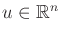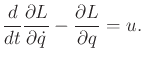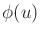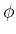### 13.4.1.3 Applying actions

Up to this point, it has been assumed that no actions are applied to the mechanical system. This is the way the Euler-Lagrange equation usually appears in physics because the goal is to predict motion, rather than control it. Letdenote an action vector. Actions can be applied to the Lagrangian formulation as generalized forces that act'' on the right side of the Euler-Lagrange equation. This results in(13.136)

The actions force the mechanical system to deviate from its usual behavior. In some instances, the true actions may be expressed in terms of other variables, and thenis obtained by a transformation (recall transforming action variables for the differential drive vehicle of Section 13.1.2). In this case,may be replaced in (13.136) byfor some transformation. In this case, the dimension ofneed not be.

Steven M LaValle 2020-08-14